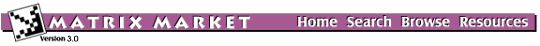## DWAVE: Square Dielectric Waveguide

### from the NEP Collection

Set DWAVE
Source: H. Dong, University of Minnesota
Discipline: Electrical engineering

Dielectric channel waveguide problems arise in many integrated circuit applications. Finite difference discretization of the governing Helmholtz equation for the magnetic field H,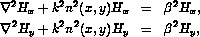,

leads to the nonsymmetric eigenvalue problem of the form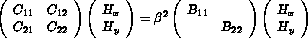where C11 and C22 are penta- or tri-diagonal matrices, C12 and C21 are (tri-)diagonal matrices, and B11 and B22 are nonsingular diagonal matrices. This generalized eigenvalue problem is reduced to a standard eigenvalue problem,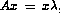, where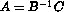, since B is diagonal.

The computational task is to determine the right most eigenvalues and their corresponding eigenvectors. In some cases, there are eigenvalues with a negative real part several orders of magnitude larger than the desired eigenvalues with positive real part. This problem presents a challenge to existing numerical methods.

Note that DWA512 and DWB512 are matrices of the same order which correspond to different parameter values.

## Matrices in this set:

The Matrix Market is a service of the Mathematical and Computational Sciences Division / Information Technology Laboratory / National Institute of Standards and Technology.

[ Home ] [ Search ] [ Browse ] [ Resources ]